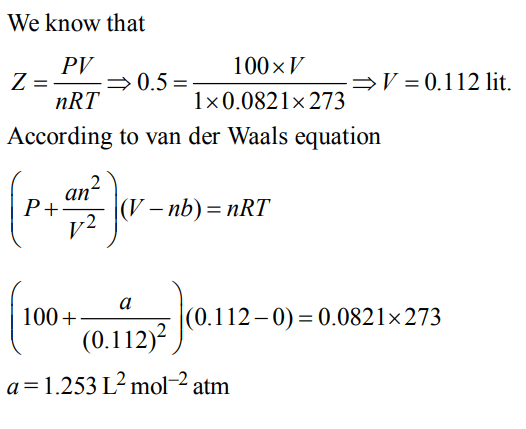## States of Matter Questions and Answers Part-9

1. Which one of the following statements is NOT true about the effect of an increase in temperature on the distribution of molecular speeds in a gas?
a) The area under the distribution curve remains the same as under the lower temperature
c) The fraction of the molecules with the most probable speed increases
d) The most probable speed increases

Explanation: As temperature rises the most probable speed increases and the fraction of molecules possessing most probable speed decreases.

2. The volume of a colloidal particle, $V_{C}$ as compared to the volume of a solute particle in a true solution $V_{S}$ , could be
a) $\frac{V_{C}}{V_{S}}\simeq10^{3}$
b) $\frac{V_{C}}{V_{S}}\simeq10^{-3}$
c) $\frac{V_{C}}{V_{S}}\simeq10^{23}$
d) $\frac{V_{C}}{V_{S}}\simeq1$

Explanation:3. Equal masses of methane and oxygen are mixed in an empty container at 25°C. The fraction of the total pressure exerted by oxygen is
a) $1\diagup2$
b) $2\diagup3$
c) $\frac{1}{3}*\frac{273}{298}$
d) $1\diagup3$

Explanation: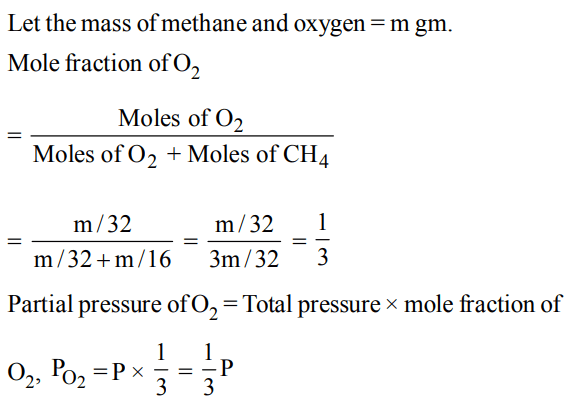4. If  $10^{-4}dm^{3}$  of water is introduced into a  $1.0dm^{3}$  flask at 300 K, how many moles of water are in the vapour phase when equilibrium is established ? (Given : Vapour pressure of  $H_{2}O$   at 300 K is 3170 Pa; $R = 8.314 J K^{-1}mol^{-1}$    )
a) $5.56× 10^{-3}mol$
b) $1.53× 10^{-2}mol$
c) $4.46× 10^{-2}mol$
d) $1.27× 10^{-3}mol$

Explanation:5. When r, P and M represent rate of diffusion, pressure and molecular mass, respectively, then the ratio of the rates of diffusion (rA / rB ) of two gases A and B, is given as :
a) $\left(P_{A}\diagup P_{B}\right)\left(M_{B}\diagup M_{A}\right)^{1\diagup 2}$
b) $\left(P_{A}\diagup P_{B}\right)^{1\diagup 2} \left(M_{A}\diagup M_{B}\right)$
c) $\left(P_{A}\diagup P_{B}\right) \left(M_{A}\diagup M_{B}\right)^{1\diagup 2}$
d) $\left(P_{A}\diagup P_{B}\right)^{1\diagup 2} \left(M_{B}\diagup M_{A}\right)$

Explanation:6. The molecular velocity of any gas is
a) inversely proportional to absolute temperature
b) directly proportional to square of temperature
c) directly proportional to square root of temperature
d) inversely proportional to the square root of temperature.

Explanation: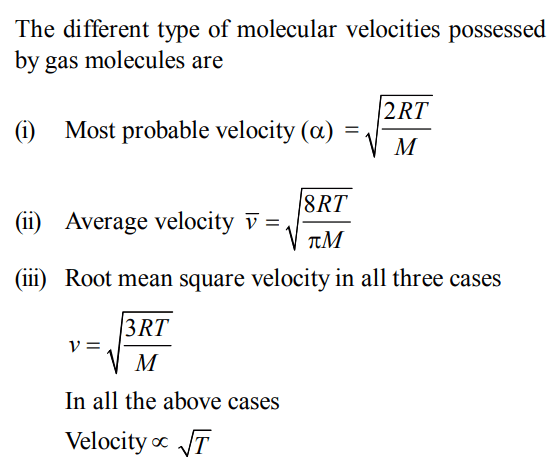7.The compressibility factor for a real gas at high pressure is :
a) $1+\frac{RT}{pb}$
b) 1
c) $1+\frac{pb}{RT}$
d) $1-\frac{pb}{RT}$

Explanation: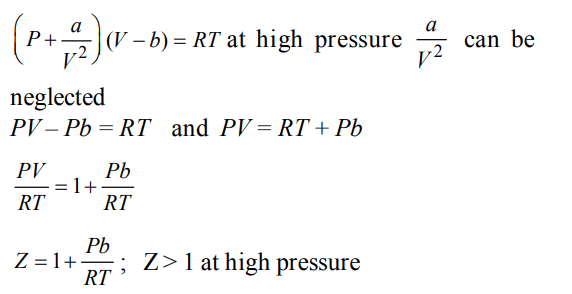8. For gaseous state, if most probable speed is denoted by C*,average speed by   $\overline{C}$  and mean square speed by C, then for a large number of molecules the ratios of these speeds are :
a) $C*:\overline{C}:C=1.225 : 1.128 : 1$
b) $C*:\overline{C}:C=1.128 : 1.225 : 1$
c) $C*:\overline{C}:C=1 : 1.128 : 1.225$
d) $C*:\overline{C}:C=1 : 1.225 : 1.128$

Explanation:9. At constant volume and temperature conditions, the rate of diffusion $D_{A}and D_{B}$   of gases A and B having densities $p_{A}and p_{B}$   are related by the expression
a) $D_{A}=\left[D_{B}\frac{P_{A}}{P_{B}}\right]^{1\diagup2}$
b) $D_{A}=\left[D_{B}\frac{P_{B}}{P_{A}}\right]^{1\diagup2}$
c) $D_{A}=D_{B}\left[\frac{P_{A}}{P_{B}}\right]^{1\diagup2}$
d) $D_{A}=D_{B}\left[\frac{P_{B}}{P_{A}}\right]^{1\diagup2}$

Explanation: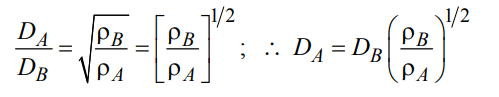10. The compression factor (compressibility factor) for 1 mole of a van der Waal’s gas at 0°C and 100 atm pressure if found to be 0.5. Assuming that the volume of gas molecules is negligible, calculate the van der Waal’s constant 'a'.
a) $0.253L^{2}mol^{-2}atm$
b) $0.53L^{2}mol^{-2}atm$
c) $1.853L^{2}mol^{-2}atm$
d) $1.253L^{2}mol^{-2}atm$